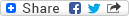#Kopule in odvisnost slučajnih spremenljivk : doktorska disertacija
ID Ružić Gorenjec, Nina (Author), ID Omladič, Matjaž (Mentor) More about this mentor...PDF - Presentation file, Download (3,40 MB)MD5: BAA249B406E72BEC919D14B5FA083A3FPID: 20.500.12556/rul/3e6463b5-b8cc-4e2e-b217-aa153f93851a

Abstract
Eden izmed možnih načinov modeliranja odvisnosti slučajnih spremenljivk vodi preko kopul, ki na ravni porazdelitvenih funkcij združujejo enorazsežne porazdelitve v večrazsežne. V doktorski disertaciji definiramo novo družino dvorazsežnih kopul, imenovano maksmin kopule, ki prav tako kot Marshallove izhajajo iz verjetnostnega modela za življenjsko dobo dvokomponentnega sistema, na katerega delujejo udari. Na sistem s komponentama ▫$A$▫ in ▫$B$▫ naj delujejo udari treh vrst. Prva vrsta udara vpliva le na komponento ▫$A$▫, druga vrsta le na komponento ▫$B$▫, tretja vrsta udara pa deluje na obe komponenti hkrati. Čase udarov zaporedoma označimo s slučajnimi spremenljivkami ▫$X$▫, ▫$Y$▫ in ▫$Z$▫ ter za njih predpostavimo, da so med seboj neodvisne. Življenjski dobi komponent ▫$A$▫ in ▫$B$▫ označimo z ▫$U$▫ oziroma z ▫$V$▫. Ker vsaka izmed komponent preneha delovati ob prvem udaru nanjo, je ▫$U = \min\{X,Z\}$▫ in ▫$V = \min\{Y,Z\}$▫. Porazdelitev ▫$(U, V)$▫ modelira Marshallova kopula. Marshall v članku [A. W. Marshall, Copulas, marginals, and joint distributions, v: L. Rüschendorf, B. Schweizer, M. D. Taylor (ur.), Distributions with Fixed Marginals and Related Topics, IMS Lecture Notes - Monograph Series, vol. 28, Institute of Mathematical Statistics, Hayward, CA, 1996, str. 213-222.] navede in dokaže izrek, ki karakterizira to družino kopul. V disertaciji pokažemo, da je za veljavnost izreka potrebno dodati še nekaj tehničnih predpostavk, ob tem pa dokažemo tudi močnejšo različico tega izreka. Če za čase udarov predpostavimo eksponentne porazdelitve, dobimo bolj znane Marshall-Olkinove kopule. Verjetnostni model Marshallovih kopul spremenimo tako, da dopuščamo možnost obnovitve komponente ▫$A$▫, medtem ko ostanejo lastnosti komponente ▫$B$▫ in treh vrst udarov nespremenjene. To si lahko predstavljamo tudi tako, da imamo na razpolago dodatni primerek komponente ▫$A$▫. Življenjska doba ▫$V = \min\{Y,Z\}$▫ komponente ▫$B$▫ ostane nespremenjena, medtem ko je ▫$U = \max\{X,Z\}$▫, saj komponenta ▫$A$▫ preneha delovati šele ob obeh udarih nanjo. Želimo poiskati slučajnima spremenljivkama ▫$U$▫ in ▫$V$▫ pripadajočo kopulo. V ta namen vpeljemo maksmin kopule, ki rešijo opisani problem. V disertaciji karakteriziramo družino maksmin kopul, raziščemo njihove lastnosti in navedemo primere.

Language: Slovenian kopula, odvisnost slučajnih spremenljivk, analiza preživetja, Marshallova kopula, Marshall-Olkinova kopula, porazdelitvena funkcija Doctoral dissertation 2.08 - Doctoral Dissertation FMF - Faculty of Mathematics and Physics Ljubljana [N. Ružić] 2015 117 str. 20.500.12556/RUL-9585751-7:519.23(043.3) 1729391324.10.2017 1299 386Copy citation## Secondary language

Language: English Copulas are one of the main tools in modelling the dependence of random variables. They join univariate distributions into the multivariate ones on the level of distribution functions. In the thesis, we define a new family of two-dimensional copulas, called maxmin copulas, which are typically applied to model the lifetime of a two-component system where components are subject to shocks, similarly as Marshall copulas. Consider a system with components ▫$A$▫ and ▫$B$▫ which are subject to three different types of shocks. The first one is fatal for Component ▫$A$▫ only, the second one for Component ▫$B$▫ only, and the third type of shock affects both components simultaneously. The independent times of occurrences of three types of shocks are denoted respectively by ▫$X$▫, ▫$Y$▫ and ▫$Z$▫. Let ▫$U$▫, respectively ▫$V$▫, denote the lifetime of Component ▫$A$▫, respectively Component ▫$B$▫. Observe that▫ $U = \min\{X,Z\}$▫ and ▫$V = \min\{Y,Z\}$▫. The distribution of ▫$(U, V )$▫ is modelled by Marshall copula. In the paper [A. W. Marshall, Copulas, marginals, and joint distributions, v: L. Rüschendorf, B. Schweizer, M. D. Taylor (ur.), Distributions with Fixed Marginals and Related Topics, IMS Lecture Notes - Monograph Series, vol. 28, Institute of Mathematical Statistics, Hayward, CA, 1996, str. 213-222.], Marshall proves a theorem that characterizes this family of copulas. In the thesis, we show that additional technical assumptions are needed, and we also prove a stronger version of this theorem. If we assume that occurences of shocks are distributed exponentially, we get well-known Marshall-Olkin copulas. We modify the above probabilistic model by allowing Component ▫$A$▫ to have a recovery option, while Component ▫$B$▫ is behaving as before. Imagine, for example, that we have an additional copy of Component ▫$A$▫. The lifetime of Component ▫$B$▫ is still expressed as ▫$V = \min\{Y,Z\}$▫, while the lifetime of Component ▫$A$▫ becomes ▫$U = \max\{X,Z\}$▫, since it is eliminated only by both types of shocks. It is our main goal to find a copula that models the lifetimes of these components. We give a full study of the augmented case by introducing maxmin copulas, which solve the described problem. In the thesis, we characterize maxmin copulas, study their properties, and give examples. copula, dependence of random variables, survival analysis, Marshall copula, Marshall-Olkin copula, distribution function

Back